coding……

# Java编程拾遗『容器——HashMap』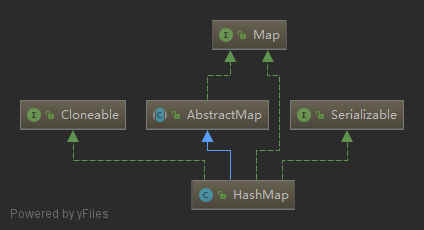## 1. 使用规则

### 1.1 构造方法

S.N. 方法 说明
1 public HashMap() 默认构造函数，为HashMap负载因子赋默认值
2 public HashMap(int initialCapacity) 构造一定容量(Node数组长度)的hashMap，由于负载因子的原因，容量不一定为initialCapacity
3 public HashMap(int initialCapacity, float loadFactor) 指定初始容量和负载因子，初始化hashMap
4 public HashMap(Map<? extends K, ? extends V> m) 通过一个Map初始化HashMap，使用默认负载因子和容量

### 1.2 Map接口

HashMap实现了Map接口，继承的抽象类AbstractMap也实现了Map接口，所以HashMap中的常用方法大多数都继承自Map接口，如下：

S.N. 方法 说明
1 void clear() 清除Map中所有的KV映射
2 default V computeIfAbsent(K key, Function<? super K, ? extends V> mappingFunction) Java 8新方法，如果key在Map中不存在，则根据Function规则计算key对应的value值，

3 default V computeIfPresent(K key,
BiFunction<? super K, ? super V, ? extends V> remappingFunction)
Java 8新方法，如果key在Map中存在，则根据BiFunction规则通过key和对应的value

4 default V compute(K key, BiFunction<? super K, ? super V, ? extends V> remappingFunction) Java 8新方法，相当于上述两个方法的综合，根据BiFunction，通过key和对应的value

5 boolean containsKey(Object key) 判断Map中是否存在key键
6 boolean containsValue(Object value) 判断Map中是否存在value值
7 Set<Map.Entry<K, V>> entrySet() 将Map所有的键值对转换为Set集合
8 boolean equals(Object o) equals方法
9 default void forEach(BiConsumer<? super K, ? super V> action) Java 8新方法，遍历Map，通过BiConsumer处理Map中每一个键值对的key、value
10 V get(Object key) 获取Map中key对应的value
11 default V getOrDefault(Object key, V defaultValue) Java 8新方法，获取Map中key对应的value，如果key不存在，则返回defaultValue
12 int hashCode() hashCode方法
13 boolean isEmpty() 判断Map是否为空
14 Set<K> keySet() 将Map中所有的key转化为Set集合
15 default V merge(K key, V value,
BiFunction<? super V, ? super V, ? extends V> remappingFunction)

BiFunction规则通过oldValue和value计算一个newValue，替换或删除(newValue为null)原

16 V put(K key, V value); 为Map添加一个键值对
17 void putAll(Map<? extends K, ? extends V> m) 将另一个Map的所有键值对添加到该Map中
18 default V putIfAbsent(K key, V value) Java 8新方法，如果Map中key不存在，则添加一个key-value键值对
19 V remove(Object key) 从Map中移除key对应的键值对
20 default boolean remove(Object key, Object value) Java8新方法，删除key-value键值对
21 default V replace(K key, V value) Java8新方法，将Map中key对应的值替换为value
22 default boolean replace(K key, V oldValue, V newValue) Java8新方法，将Map中key-oldValue键值对的value值替换为newValue
23 default void replaceAll(BiFunction<? super K, ? super V, ? extends V> function) Java8新方法，将Map中所有的键值对的value，替换为根据BiFunction规则通过key-value

24 int size() 返回Map中EntrySet的数目
25 Collection<V> values() 返回Map中所有value组成的集合

## 2. 实现源码分析``````static class Node<K,V> implements Map.Entry<K,V> {
final int hash;
final K key;
V value;
Node<K,V> next;

Node(int hash, K key, V value, Node<K,V> next) {
this.hash = hash;
this.key = key;
this.value = value;
this.next = next;
}

public final K getKey()        { return key; }
public final V getValue()      { return value; }
public final String toString() { return key + "=" + value; }

public final int hashCode() {
return Objects.hashCode(key) ^ Objects.hashCode(value);
}

public final V setValue(V newValue) {
V oldValue = value;
value = newValue;
return oldValue;
}

public final boolean equals(Object o) {
if (o == this)
return true;
if (o instanceof Map.Entry) {
Map.Entry<?,?> e = (Map.Entry<?,?>)o;
if (Objects.equals(key, e.getKey()) &&
Objects.equals(value, e.getValue()))
return true;
}
return false;
}
}``````

Node是HashMap的一个内部类，实现了Map.Entry接口，本质是就是一个映射(键值对)，上图中的每个黑色圆点就是一个Node对象。除了key和value，Node中还有两个成员变量hash和一个自身类型的引用next，分别表示key的哈希值及当前Node节点的相邻节点引用(组织链表)。

HashMap就是使用哈希表来存储的。哈希表为解决冲突，可以采用开放地址法和链地址法等来解决问题，Java中HashMap采用了链地址法。链地址法，简单来说，就是数组加链表的结合。在每个数组元素上都一个链表结构，当数据被Hash后，得到数组下标，把数据放在对应下标元素的链表上。例如程序执行下面代码：

``map.put("zhuo", 1);``

``````transient Node<K,V>[] table; //Map中用来存储key-value对Node节点的数组
int threshold;             // 所能容纳的key-value对极限
int modCount;  //修改次数
int size;     //Map中key-value对数目``````

size这个字段其实很好理解，就是HashMap中实际存在的键值对数量。注意和table的长度length、容纳最大键值对数量threshold的区别。而modCount字段主要用来记录HashMap内部结构发生变化的次数，主要用于迭代的快速失败(ConcurrentModificationException)。强调一点，内部结构发生变化指的是结构发生变化，例如put新键值对，但是某个key对应的value值被覆盖不属于结构变化

### 2.1 构造函数

``````public HashMap() {
}``````

``````public HashMap(int initialCapacity, float loadFactor) {
if (initialCapacity < 0)
throw new IllegalArgumentException("Illegal initial capacity: " +
initialCapacity);
if (initialCapacity > MAXIMUM_CAPACITY)
initialCapacity = MAXIMUM_CAPACITY;
throw new IllegalArgumentException("Illegal load factor: " +
this.threshold = tableSizeFor(initialCapacity);
}``````

``Map<Stirng, Integer> strMap = new HashMap(13, 0.75);``

``````public HashMap() {
}``````
``````public HashMap(int initialCapacity, float loadFactor) {
threshold = initialCapacity;
}``````

``````public HashMap(int initialCapacity) {
}``````

``````public HashMap(Map<? extends K, ? extends V> m) {
putMapEntries(m, false);
}``````

``````/*
* 将Map中的键值对插入到当前HashMap中
*/
final void putMapEntries(Map<? extends K, ? extends V> m, boolean evict) {
int s = m.size();
//s > 0，说明Map不为空，依次将Map中的键值对插入到当前HashMap中
if (s > 0) {
if (table == null) { // pre-size
//table == null，说明当前HashMap还没进行过插入操作
//则对根据s的大小，初始化当前HashMap的threshold，使其能够容纳m

//ft即为能够容纳m的hash桶数组length的最小值(因为数组长度必须为2的整数幂，所以ft不一定为最终值)
float ft = ((float)s / loadFactor) + 1.0F;

//检查ft是否超过最大值，如果超过了就赋最大值
int t = ((ft < (float)MAXIMUM_CAPACITY) ?
(int)ft : MAXIMUM_CAPACITY);

//调用上述构造函数，threshold未进行赋值，所以为0，肯定小于t
if (t > threshold)
//threshold为大于t的最小的2的整数次幂，这个threshold不是最终的threshold，
threshold = tableSizeFor(t);
}
//如果table不为null，并且s大于当前HashMap的threshold，进行扩容
else if (s > threshold)
resize();
//遍历Map，调用putVal插入Map中的键值对
for (Map.Entry<? extends K, ? extends V> e : m.entrySet()) {
K key = e.getKey();
V value = e.getValue();
putVal(hash(key), key, value, false, evict);
}
}
}``````

``````s/0.75 + 1 < 2^n

### 2.2 put方法

Java8中put操作如下所示：

``````public V put(K key, V value) {
// 对key的hashCode()做hash
return putVal(hash(key), key, value, false, true);
}
``````
``````final V putVal(int hash, K key, V value, boolean onlyIfAbsent,
boolean evict) {
Node<K,V>[] tab; Node<K,V> p; int n, i;
// 1：tab为空则创建
if ((tab = table) == null || (n = tab.length) == 0)
n = (tab = resize()).length;
// 2：计算index，并对null做处理
if ((p = tab[i = (n - 1) & hash]) == null)
tab[i] = newNode(hash, key, value, null);
else {
Node<K,V> e; K k;
// 3：节点key存在，直接覆盖value
if (p.hash == hash &&
((k = p.key) == key || (key != null && key.equals(k))))
e = p;
// 4：判断该链为红黑树
else if (p instanceof TreeNode)
e = ((TreeNode<K,V>)p).putTreeVal(this, tab, hash, key, value);
// 5：该链为链表
else {
for (int binCount = 0; ; ++binCount) {
if ((e = p.next) == null) {
p.next = newNode(hash, key,value,null);
//链表长度大于8转换为红黑树进行处理
if (binCount >= TREEIFY_THRESHOLD - 1) // -1 for 1st
treeifyBin(tab, hash);
break;
}
// key已经存在直接覆盖value
if (e.hash == hash &&
((k = e.key) == key || (key != null && key.equals(k))))
break;
p = e;
}
}

if (e != null) { // existing mapping for key
V oldValue = e.value;
if (!onlyIfAbsent || oldValue == null)
e.value = value;
afterNodeAccess(e);
return oldValue;
}
}

++modCount;
// 6：超过最大容量 就扩容
if (++size > threshold)
resize();
afterNodeInsertion(evict);
return null;
}``````
``````final void treeifyBin(Node<K,V>[] tab, int hash) {
int n, index; Node<K,V> e;
if (tab == null || (n = tab.length) < MIN_TREEIFY_CAPACITY)
//如果数组长度小于64，进行扩容，并不会转化为红黑树
resize();
else if ((e = tab[index = (n - 1) & hash]) != null) {
//数组长度大于64，将链表转化为红黑树
TreeNode<K,V> hd = null, tl = null;
do {
TreeNode<K,V> p = replacementTreeNode(e, null);
if (tl == null)
hd = p;
else {
p.prev = tl;
tl.next = p;
}
tl = p;
} while ((e = e.next) != null);
if ((tab[index] = hd) != null)
hd.treeify(tab);
}
}``````1. 判断键值对数组table[i]是否为空或为null，否则执行resize()进行扩容
2. 根据键值key计算hash值得到插入的数组索引i，如果table[i]==null，直接新建节点添加，转向6，如果table[i]不为空，则转向3
3. 判断table[i]的首个元素是否和key一样，如果相同直接覆盖value，否则转向4，这里的相同指的是hashCode以及equals
4. 判断table[i] 是否为treeNode，即table[i] 是否是红黑树，如果是红黑树，则直接在树中插入键值对，否则转向5
5. 遍历table[i]，判断链表长度是否大于8，大于8且数组length大于64的话把链表转换为红黑树，在红黑树中执行插入操作，否则对数组进行扩容，进行链表的插入操作；遍历过程中若发现key已经存在直接覆盖value即可
6. 插入成功后，判断实际存在的键值对数量size是否超多了最大容量threshold，如果超过，进行扩容

• Java8 HashMap是数组+链表+红黑树组织的，之前的版本时通过数组+链表组织的。在Java8中如果链表长度大于8并且数组length大于64，会转化成红黑树
• 对于链表结构，在进行put操作，向某个链表中添加元素时，Java8使用的时尾插法，而Java8之前的版本使用的是头插法
• 计算key的哈希值方法不同，Java8中优化了高位运算的算法，通过hashCode()的高16位异或低16位实现的
• resize实现不同，Java8中resize不会产生环链，从而导致cpu使用率飙升到100%，但仍有数据丢失的可能。另外Java8之前的版本，旧链表迁移新链表的时候，如果在新表的数组索引位置相同，则链表元素会倒置，而Java8会保持原来链表的顺序。

#### 2.2.1 确定哈希桶数组索引位置

``````//方法一：
static final int hash(Object key) { //java7&java8
int h;
// h = key.hashCode() 为第一步 取hashCode值
// h ^ (h >>> 16)  为第二步 高位参与运算
return (key == null) ? 0 : (h = key.hashCode()) ^ (h >>> 16);
}

//方法二：
static int indexFor(int h, int length) {  //java7源码，java8中没有这个方法，但是实现原理一样的
return h & (length-1);  //第三步 取模运算
}``````

####2.2.2 扩容机制

``````final Node<K,V>[] resize() {
Node<K,V>[] oldTab = table;
int oldCap = (oldTab == null) ? 0 : oldTab.length;
int oldThr = threshold;
int newCap, newThr = 0;
if (oldCap > 0) {
// 超过最大值就不再扩充了，就只好随你碰撞去吧
if (oldCap >= MAXIMUM_CAPACITY) {
threshold = Integer.MAX_VALUE;
return oldTab;
}
// 没超过最大值，就扩充为原来的2倍
else if ((newCap = oldCap << 1) < MAXIMUM_CAPACITY &&
oldCap >= DEFAULT_INITIAL_CAPACITY)
newThr = oldThr << 1; // double threshold
}
else if (oldThr > 0) // initial capacity was placed in threshold
newCap = oldThr;
else {               // zero initial threshold signifies using defaults
newCap = DEFAULT_INITIAL_CAPACITY;
}
// 计算新的resize上限
if (newThr == 0) {

float ft = (float)newCap * loadFactor;
newThr = (newCap < MAXIMUM_CAPACITY && ft < (float)MAXIMUM_CAPACITY ?
(int)ft : Integer.MAX_VALUE);
}
threshold = newThr;
@SuppressWarnings({"rawtypes"，"unchecked"})
Node<K,V>[] newTab = (Node<K,V>[])new Node[newCap];
table = newTab;
if (oldTab != null) {
// 把每个bucket都移动到新的buckets中
for (int j = 0; j < oldCap; ++j) {
Node<K,V> e;
if ((e = oldTab[j]) != null) {
oldTab[j] = null;
if (e.next == null)
newTab[e.hash & (newCap - 1)] = e;
else if (e instanceof TreeNode)
((TreeNode<K,V>)e).split(this, newTab, j, oldCap);
else { // 链表优化重hash的代码块
Node<K,V> loHead = null, loTail = null;
Node<K,V> hiHead = null, hiTail = null;
Node<K,V> next;
do {
next = e.next;
// 原索引
if ((e.hash & oldCap) == 0) {
if (loTail == null)
else
loTail.next = e;
loTail = e;
}
// 原索引+oldCap
else {
if (hiTail == null)
else
hiTail.next = e;
hiTail = e;
}
} while ((e = next) != null);
// 原索引放到bucket里
if (loTail != null) {
loTail.next = null;
}
// 原索引+oldCap放到bucket里
if (hiTail != null) {
hiTail.next = null;
}
}
}
}
}
return newTab;
}``````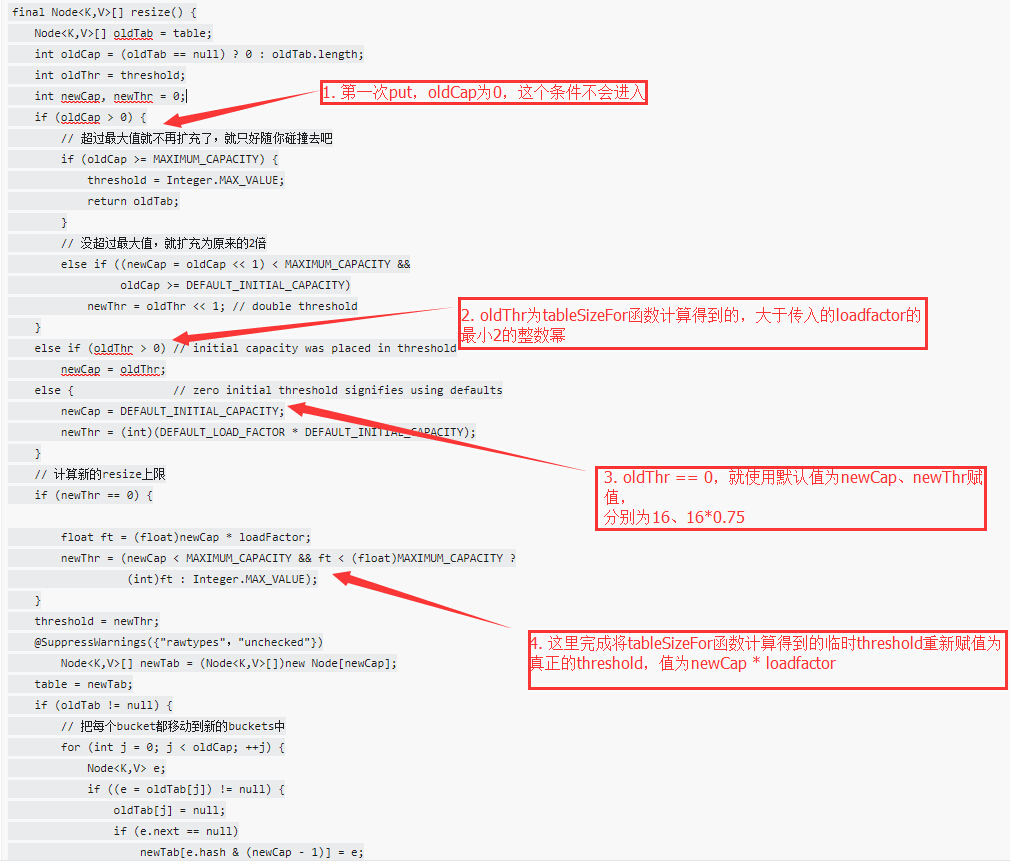``````public V put(K key, V value) {
if (table == EMPTY_TABLE) {
inflateTable(threshold);
}
if (key == null)
return putForNullKey(value);
int hash = hash(key);
int i = indexFor(hash, table.length);
for (Entry<K,V> e = table[i]; e != null; e = e.next) {
Object k;
if (e.hash == hash && ((k = e.key) == key || key.equals(k))) {
V oldValue = e.value;
e.value = value;
e.recordAccess(this);
return oldValue;
}
}

modCount++;
return null;
}  ``````
``````void addEntry(int hash, K key, V value, int bucketIndex) {
if ((size >= threshold) && (null != table[bucketIndex])) {
resize(2 * table.length);
hash = (null != key) ? hash(key) : 0;
bucketIndex = indexFor(hash, table.length);
}

createEntry(hash, key, value, bucketIndex);
}``````
``````void resize(int newCapacity) {   //传入新的容量
Entry[] oldTable = table;    //引用扩容前的Entry数组
int oldCapacity = oldTable.length;
if (oldCapacity == MAXIMUM_CAPACITY) {  //扩容前的数组大小如果已经达到最大(2^30)了
threshold = Integer.MAX_VALUE; //修改阈值为int的最大值(2^31-1)，这样以后就不会扩容了
return;
}

Entry[] newTable = new Entry[newCapacity];  //初始化一个新的Entry数组
transfer(newTable);                         //！！将数据转移到新的Entry数组里
table = newTable;                           //HashMap的table属性引用新的Entry数组
}``````
``````void transfer(Entry[] newTable) {
Entry[] src = table;                   //src引用了旧的Entry数组
int newCapacity = newTable.length;
for (int j = 0; j < src.length; j++) { //遍历旧的Entry数组
Entry<K,V> e = src[j];             //取得旧Entry数组的每个元素
if (e != null) {
src[j] = null;//释放旧Entry数组的对象引用（for循环后，旧的Entry数组不再引用任何对象）
do {
Entry<K,V> next = e.next;
int i = indexFor(e.hash, newCapacity); //！！重新计算每个元素在数组中的位置
e.next = newTable[i]; //标记
newTable[i] = e;      //将元素放在数组上
e = next;             //访问下一个Entry链上的元素
} while (e != null);
}
}
}``````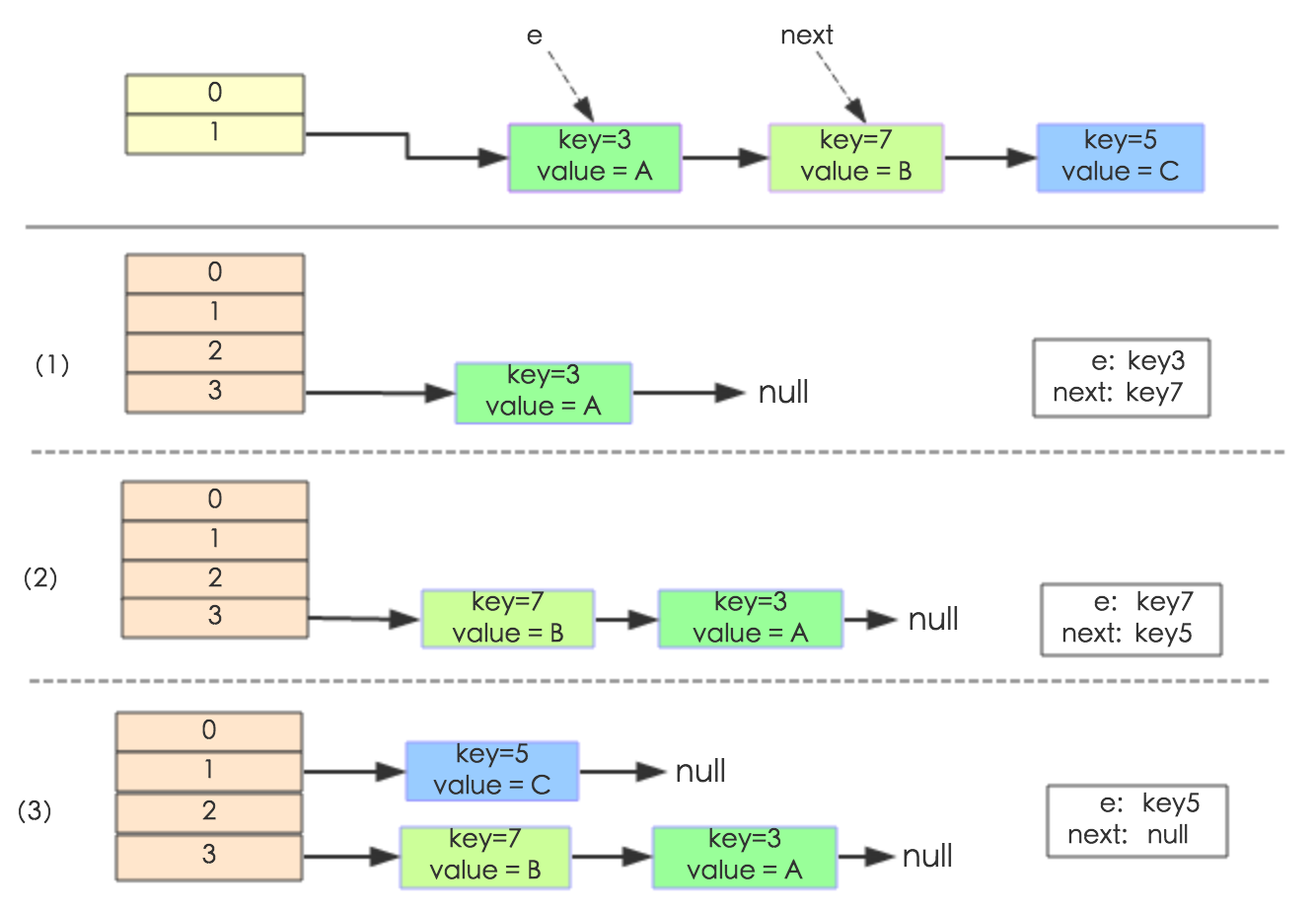下面讲解下Java8做了哪些优化。经过观测可以发现，我们使用的是2次幂的扩展(指长度扩为原来2倍)，所以，元素的位置要么是在原位置，要么是在原位置再移动2次幂的位置。看下图可以明白这句话的意思，n为table的长度，图（a）表示扩容前的key1和key2两种key确定索引位置的示例，图（b）表示扩容后key1和key2两种key确定索引位置的示例，其中hash1是key1对应的哈希与高位运算结果。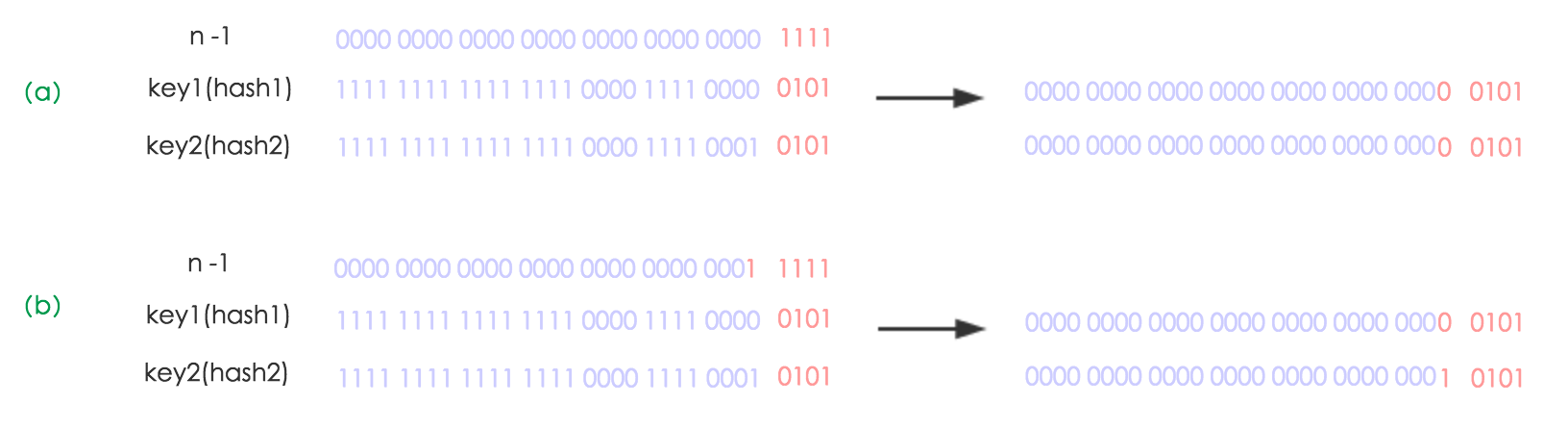元素在重新计算hash之后，因为n变为2倍，那么n-1的mask范围在高位多1bit(红色)，因此新的index就会发生这样的变化：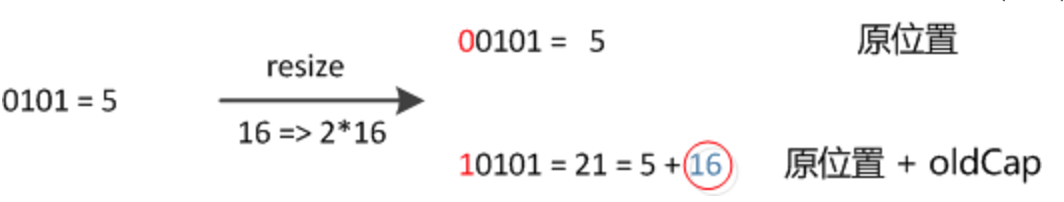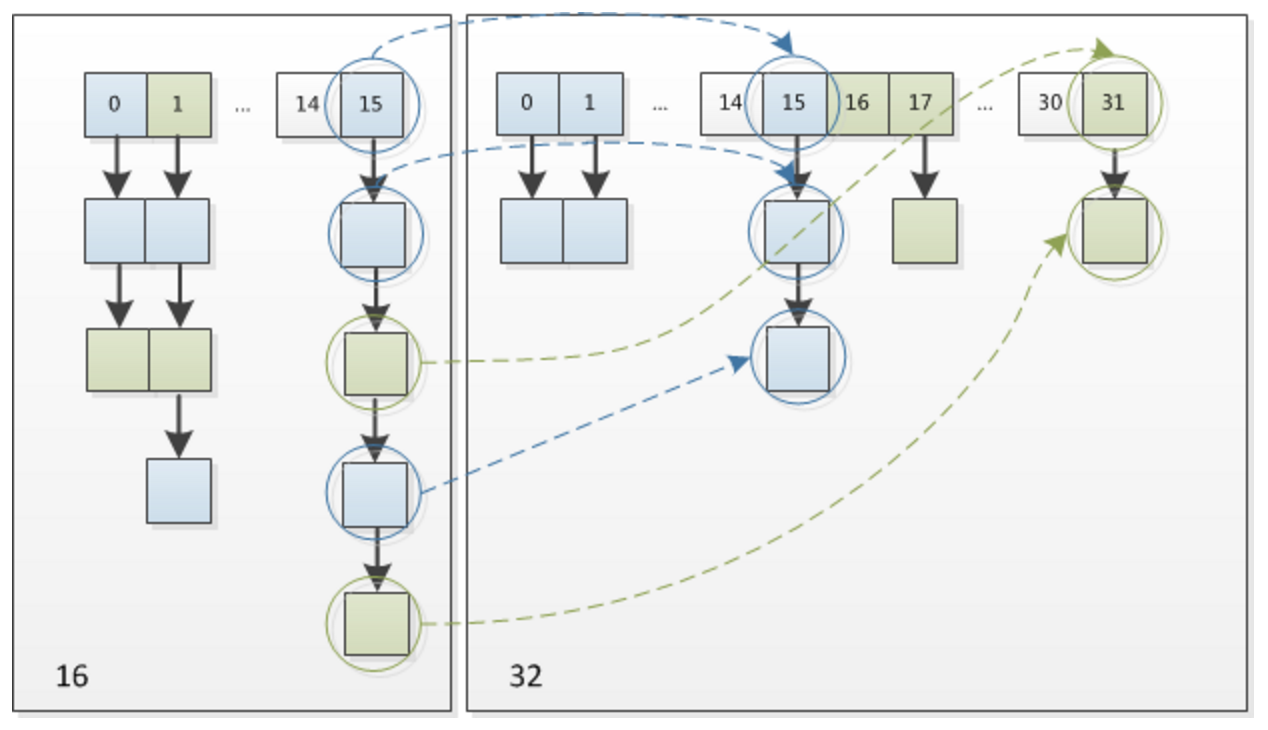这个设计确实非常的巧妙，既省去了重新计算hash值的时间，而且同时，由于新增的1bit是0还是1可以认为是随机的，因此resize的过程，均匀的把之前的冲突的节点分散到新的bucket了。这一块就是Java8新增的优化点。有一点注意区别，Java7中rehash的时候，旧链表迁移新链表的时候，如果在新表的数组索引位置相同，则链表元素会倒置，但是从上图可以看出，Java8中不会倒置

### 2.3 线程安全

HashMap在多线程环境中时不安全的，在多线程使用场景中，应该尽量避免使用线程不安全的HashMap，而使用线程安全的ConcurrentHashMap。而我们取查询为什么HashMap在多线程下不安全，得到的答案总是：多线程场景下，HashMap可能造成死循环，导致cpu使用率飙升到100%。这里先将一下结论，其实HashMap在Java8之前的版本，多线程场景下会造成死循环，并且有可能会丢失数据，但是Java8之后，不会再造成死循环，但是无法规避数据丢失的可能。下面首先来看一下Java7 HashMap再多线程场景下为什么会造成死循环。

``````public class HashMapInfiniteLoop {

private static HashMap<Integer,String> map = new HashMap<Integer,String>(2，0.75f);
public static void main(String[] args) {
map.put(5， "C");

public void run() {
map.put(7, "B");
System.out.println(map);
};
}.start();
public void run() {
map.put(3, "A);
System.out.println(map);
};
}.start();
}
}``````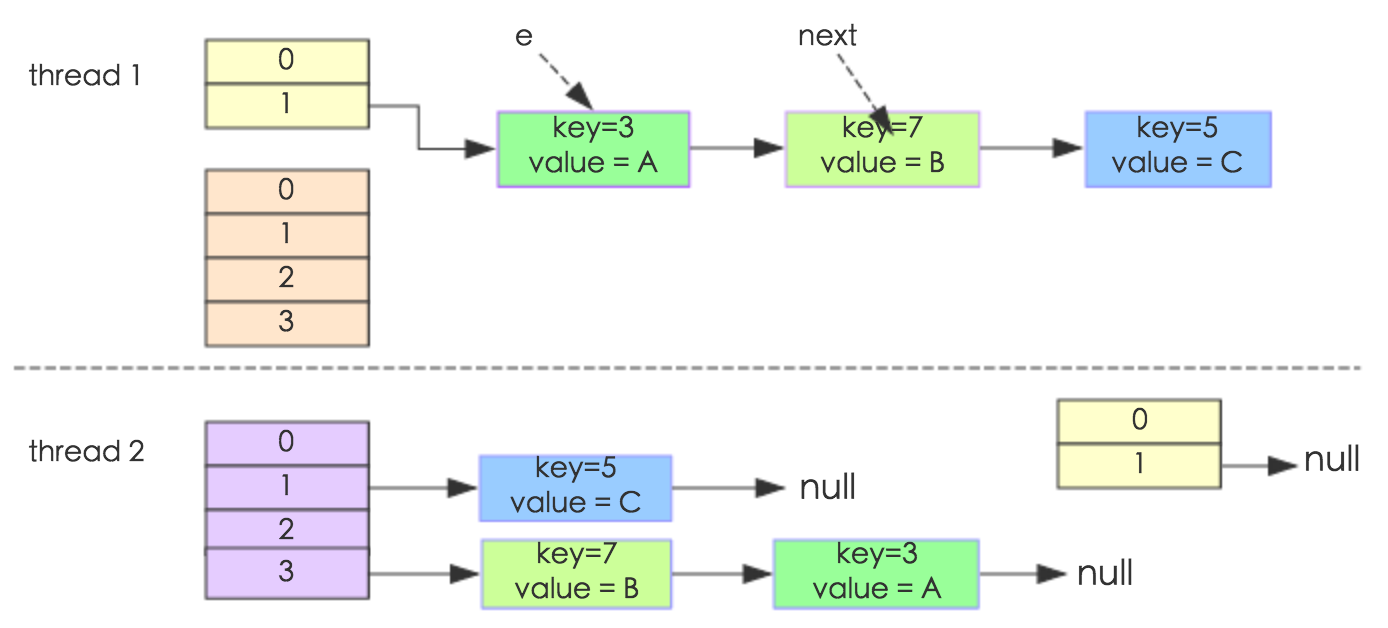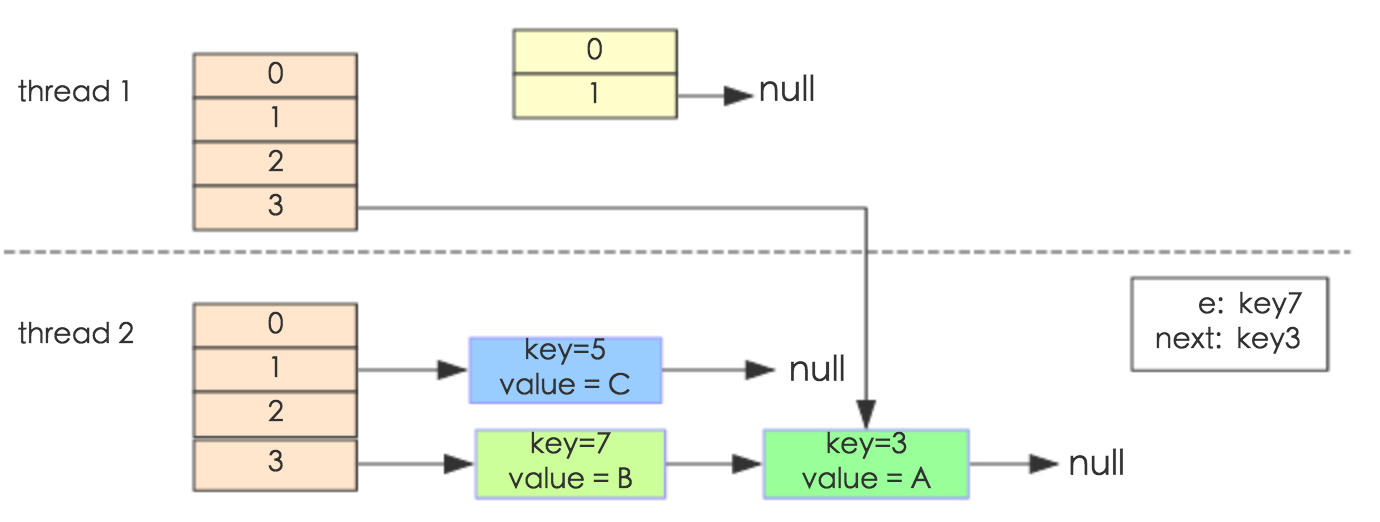e.next = newTable[i] 导致 key(3).next 指向了 key(7)。注意：此时的key(7).next 已经指向了key(3)， 环形链表就这样出现了。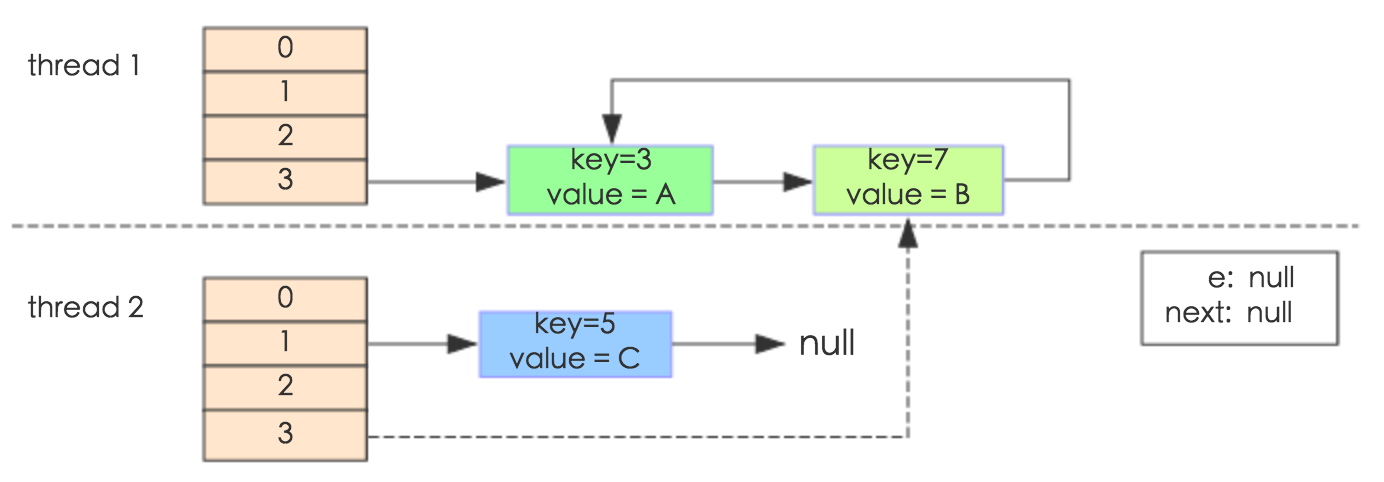### 2.4 get(Object key)方法

``````public V get(Object key) {
Node<K,V> e;
return (e = getNode(hash(key), key)) == null ? null : e.value;
}``````
``````final Node<K,V> getNode(int hash, Object key) {
Node<K,V>[] tab; Node<K,V> first, e; int n; K k;
//获取key所在hash桶数组索引的第一个元素first
if ((tab = table) != null && (n = tab.length) > 0 &&
(first = tab[(n - 1) & hash]) != null) {
if (first.hash == hash && // 比较第一个元素first与key是否相等
((k = first.key) == key || (key != null && key.equals(k))))
return first;
//第一个元素不相等，遍历first的后继元素
if ((e = first.next) != null) {
if (first instanceof TreeNode)
//如果位红黑树，则取遍历红黑树，获取与key相等的TreeNode节点
return ((TreeNode<K,V>)first).getTreeNode(hash, key);
do {
//遍历链表，获取与key相等的Node节点
if (e.hash == hash &&
((k = e.key) == key || (key != null && key.equals(k))))
return e;
} while ((e = e.next) != null);
}
}
return null;
}``````

### 2.5 containsKey(Object key)方法

``````public boolean containsKey(Object key) {
return getNode(hash(key), key) != null;
}``````

### 2.6 containsKey(Object key)方法

``````public boolean containsValue(Object value) {
Node<K,V>[] tab; V v;
if ((tab = table) != null && size > 0) {
//遍历哈希桶数组每个元素
for (int i = 0; i < tab.length; ++i) {
//遍历哈希桶数组各个index位置的元素
for (Node<K,V> e = tab[i]; e != null; e = e.next) {
if ((v = e.value) == value ||
(value != null && value.equals(v)))
return true;
}
}
}
return false;
}``````

### 2.7 remove(Object key)方法

``````public V remove(Object key) {
Node<K,V> e;
return (e = removeNode(hash(key), key, null, false, true)) == null ?
null : e.value;
}``````

``````/**
* @param hash key的哈希值
* @param key key
* @param value 如果matchValue为true，则比较value，否则忽略value
* @param matchValue 如果为true，只有当value也相等时，才删除节点
* @param movable 如果为false，在删除时，不移动其他Node，作用于红黑树节点删除
* @return 返回删除的Node，如果未删除，返回null
*/
final Node<K,V> removeNode(int hash, Object key, Object value,
boolean matchValue, boolean movable) {
Node<K,V>[] tab; Node<K,V> p; int n, index;
if ((tab = table) != null && (n = tab.length) > 0 &&
(p = tab[index = (n - 1) & hash]) != null) {
//与getNode(int hash, Object key)一致，获取key对应的Node
Node<K,V> node = null, e; K k; V v;
if (p.hash == hash &&
((k = p.key) == key || (key != null && key.equals(k))))
node = p;
else if ((e = p.next) != null) {
if (p instanceof TreeNode)
node = ((TreeNode<K,V>)p).getTreeNode(hash, key);
else {
do {
if (e.hash == hash &&
((k = e.key) == key ||
(key != null && key.equals(k)))) {
node = e;
break;
}
p = e;
} while ((e = e.next) != null);
}
}
//判断是否能删除Node
if (node != null && (!matchValue || (v = node.value) == value ||
(value != null && value.equals(v)))) {
if (node instanceof TreeNode)
//红黑树节点删除处理
((TreeNode<K,V>)node).removeTreeNode(this, tab, movable);
else if (node == p)
//node为链表首节点，将tab[index]指向node的后继节点
tab[index] = node.next;
else
//node非首节点，删除node节点，p为node的前置节点，p的next指针指向node的next后继节点
p.next = node.next;
++modCount;
--size;
afterNodeRemoval(node);
return node;
}
}
return null;
}``````

### 2.8 remove(Object key, Object value)方法

``````public boolean remove(Object key, Object value) {
return removeNode(hash(key), key, value, true, true) != null;
}``````

### 3. HashMap特点

• 根据键保存和获取值的效率都很高，为O(1)
• HashMap中的键值对没有顺序，因为hash值是随机的

### 评论 2

1. #1

楼主，环链分析那里感觉不对啊，不应该是先数据转移transfer()，再createEntry()的吗，第一张图中的table怎么会有3个节点呢。
我这里引用了楼主的文章，稍作修改。。

白菜饼5年前 (2019-03-20)回复
• 看了一下，确实有问题，环链这一段是美团技术博客分享的，分析的出发点是table有三个节点，这种情况下确实会产生环链。但是事实上应该只有节点5一个节点，这一块还要仔细分析一下，多谢指正，可以的话把文章链接给一下，学习一下

zhuoli5年前 (2019-03-22)回复
• QQ咨询
• 回顶